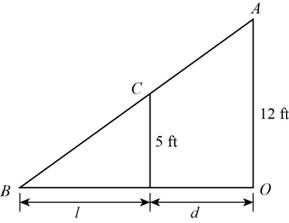# The function that models the length ( L ) of shadow of woman standing near a street lamp in terms of her distance ( d ) from the base of the lamp.### Precalculus: Mathematics for Calcu...

6th Edition
Stewart + 5 others
Publisher: Cengage Learning
ISBN: 9780840068071### Precalculus: Mathematics for Calcu...

6th Edition
Stewart + 5 others
Publisher: Cengage Learning
ISBN: 9780840068071

#### Solutions

Chapter 2, Problem 12P
To determine

## To find: The function that models the length (L) of shadow of woman standing near a street lamp in terms of her distance (d) from the base of the lamp.

Expert Solution

The function that models L   in terms of d is L(d)=57d,d>0 .

### Explanation of Solution

The geometric figure of the situation is shown below,Figure (1)

In triangle AOB as shown in Figure (1), OA is height of street lamp, CD is height of woman, L is the length of shadow of woman and d is the distance of woman from base of street lamp.

Height of woman is 5ft . and height of street lamp is 12ft

Triangles AOB and CDB are similar and corresponding sides of similar triangles are in same proportion.

Mathematically,

CDAO=BDBO

Substitute 5 for CD, 12 for AO, L for BD and BO for L+d in above equation.